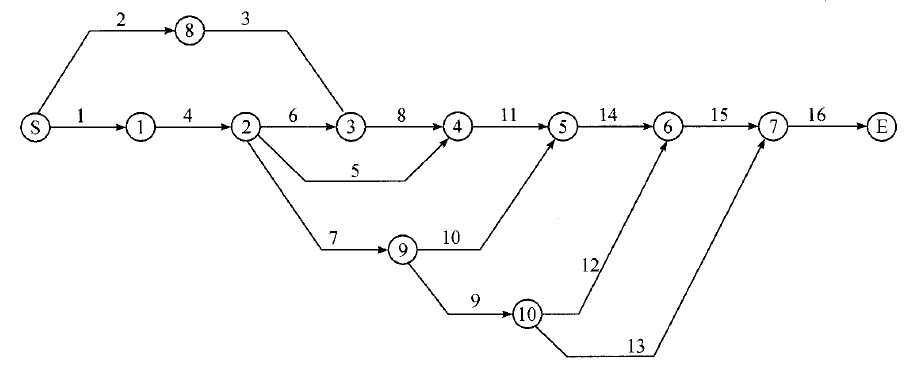# 商业伙伴挑选问题

Partner selection is an important problem in agile manufacturing and supply chain management. We try to solve it by gene algorithm with embedded fuzzy rules.

1) Problem description

Suppose a company won a bid of a big project, which consists of $n$ sub projects. The company can not finish the project by itself, so it must invite bids for sub projects. The relationship of these sub projects composes an activity network. Let $(i,k)\in{H}$ stand for that project $i$ should be finished before project $k$ started, where $H$ is the set of all the cohensive relationships. The investment cash flow is $e(t)\ge{0}$, $t=1,\cdots,D$, where $D$ is the completion day of the whole project. If the project is delayed, the company will be penalized with money number $\beta$ per unit time.

Suppose for project $i$, $i=1,\cdots,n$, there are $m_i$ candidate bidders. $b_{ij}$ is the bid price of bidder $j$ of project $i$, and the completion time is $q_{ij}$. The company will pay $\alpha{b_{ij}}(0\le{\alpha\le{1}})$ before the sub project is started, and the remaining $(1-\alpha)b_{ij}$ will be paid after the sub project is completed. When the company falls short of money, it can lend money from banks with interest rate $r$.

The goal of the company is to select the optimal partner combination in order to minimize the cost consists of expense of sub projects, penalty of project delay and the bank interest.

2) Math model

Define

$w_{ij}(t)=\begin{cases}1,\ bidder\ j\ of\ sub\ project\ i\ take\ the\ project\ starting\ at\ t\\0,\ else\end{cases}$

So the problem can be modeled as

$\mathop{min}_wZ(w)=\sum\limits_{i=1}^n{\sum\limits_{j=1}^{m_i}b_{ij}\sum\limits_{t=1}^{c_n}w_{ij}(t)}+r\sum\limits_{t=1}^{c_n}[\alpha{\sum\limits_{i=1}^n{\sum\limits_{j=1}^{m_i} \sum\limits_{\tau=1}^{t}b_{ij}w_{ij}(\tau)}}+$

$(1-\alpha){\sum\limits_{i=1}^n{\sum\limits_{j=1}^{m_i}\sum\limits_{\tau=1}^{t}b_{ij}w_{ij}(\tau-q_{ij})}}-\sum\limits_{\tau=1}^te(\tau)]^{+}+\beta[c_n-D]^{+}$

$s.t.\ \sum\limits_{j=1}^{m_i}\sum\limits_{t=1}^{c_n}w_{ij}(t)=1,\ i=1,\cdots,n$

$\sum\limits_{j=1}^{m_i}\sum\limits_{t=1}^{c_n}(t+q_{ij})w_{ij}(t)\le{\sum\limits_{j=1}^{m_k}\sum\limits_{t=1}^{c_n}tw_{kj}(t)},\forall\ (i,k)\in{H}$

$\sum\limits_{j=1}^{m_n}\sum\limits_{t=1}^{c_n}(t+q_{nj})w_{nj}(t)=c_n$

$w_{ij}(t)=1\ or\ 0, \forall{i,j,t}$

where $c_n$ is the completion time of the project, $[x]^{+}$ stands for $max\{0,x\}$.

The target function of the above model is not continuously differentiable, the ordinary mathematical programming can not solve the problem.

If the delay penalty factor $\beta$ is too small, the company will not lend money from the bank by delaying the project. So there must be a condition such that the problem is an advance/delay penalty problem. The solution to an advance/delay penalty problem is said to be a regular solution. Set the total investment for the project is $E=\sum\limits_{t=1}^De(t)$. The following theorem gives the sufficient condition if there exists a regular solution of the problem.

Theorem 1: If $\beta\ge{rE}$, the problem has a regular solution.

Proof is ommited here.

3) Implementation

The solution space of the problem is $N=\prod\limits_{i=1}^nm_i$. It is very big even for a small size problem. The following definition and theorem will help reduce the solution space.

Definition 1: Candidate $j$ of sub project $i$ is invalid, if there exists another candidate $k$ of sub project $i$, such that $b_{ik}\le{b_{ij}}$, $q_{ik}< q_{ij}$ or $b_{ik}< b_{ij}$, $q_{ik}\le{q_{ij}}$.

Theorem 2: If the model has regular solutions, then at least there exists one optimal solution with no invalid candidates.

For sub project $i$, sort the bidders with bid price from low to high,

$b_{i1} < b_{i2}< \cdots < b_{im_i}, i=1,\cdots,n,$

delete all invalid bidders, we have

$q_{i1} > q_{i2} > \cdots > q_{im_i}, i=1,\cdots,n,$

By definition 1, there is no pair of candidates with the same bid price or the same project time. Cadidates with the same bid price and the same project time could not be identified. So the two inequalities above are strict.

Solve the problem by gene algorithm. Set the chromosome $x=[x_1,\cdots,x_n]$, $x_i\in[1,m_i]$ and is a natural number, standing for the candidate $x_i$ is selected for sub project $i$. Set $s_i(x)$ and $c_i(x)$ the start time and completion time of sub project $i$ in the selection $x$. The model can be rewritten as

$\mathop{min}_xZ(x)=\sum\limits_{i=1}^nb_{ix_i}+r\sum\limits_{t=1}^{c_n{x}}[\alpha\sum\limits_{s_i(x)\le{t}}b_{ix_i}+(1-\alpha)\sum\limits_{c_i(x)\le{t}}b_{ix_i}-\sum\limits_{\tau=1}^te(\tau)]^{+}+\beta[c_n(x)-D]^{+}$

$s.t. c_i(x)\le{s_k(x)},\forall{(i,k)\in{H}}$

$x_i\in{[1,m_i]},x_i\in{N},i=1,\cdots,n$

The above model is simpler, but the subscript contains variables, which can not be solved by mathematical programming. Gene algorithm can solve this.

For a given selection $x$, the total completion time of the project can be obetained by critical path method(CPM). By CPM we can get the target value of the problem and the critical project and non-critical project. Note that for different selection of $x$, the critical path is different.

Solutions can be improved by fuzzy decision. The basic idea is as follows. When the selection results in the delay of the project, the sub projects on the critical path should assigned to a candidate with higher bid price. If the total fee of the project is high, the sub projects on the non-critical path should be assigned to the candidates with lower bid price.

We define the fitness function

$f(j)=Z_{max}-Z(j)+a,j=1,\cdots,NP,$

where $NP$ is the size of population, $Z_{max}=max\{Z(x(j)),j=1,\cdots,NP\}$ is the largest target value of the population. $a$ is a tuning parameter which makes the chromosome with largest target value have the chance to have offsprings. $a$ is updated with $a=0.975a$ with initial value $5\%$ of the largest target value of the initial population.

Gene operation is implemented with crossover with two cut points and mutation with value changes. As long as the selection for sub project $i$ is in $[1,m_i]$, the selection is reasonable.

Here is a real example from a thermal power station. The project consists of 16 sub projects with completion time 36 months. The delay penalty for a month is 48 million Yuan. The payment plan is $65\%$ when the sub project is started and the remaining is paid after the completion. The interest rate of bank is $0.6\%$ per month. The total investment is 485 million Yuan.

The precedence relationship is represented by the image:The optimal solution obtained from gene algorithm is 472.4842 million Yuan, and the completion time is 36 months. The company running the project will profit about 12.52 million Yuan.